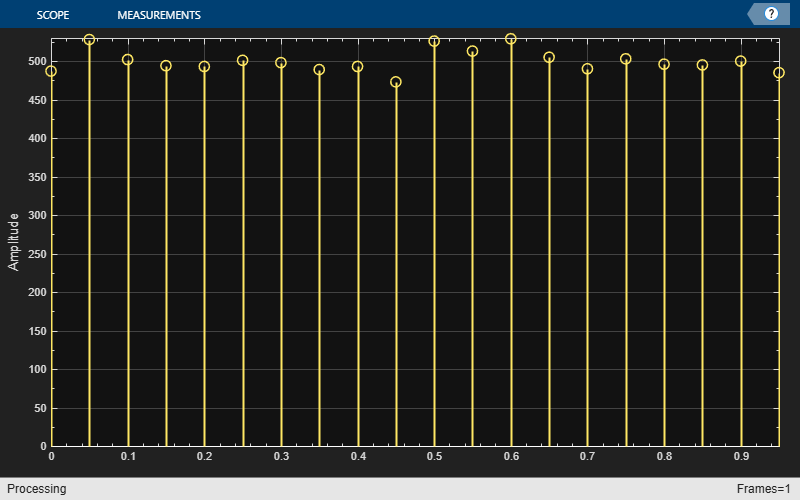# Visualize Central Limit Theorem in Array Plot

This example shows how to use and configure the `dsp.ArrayPlot` System object to visualize the Central Limit Theorem. This theorem states that if you take a large number of random samples from a population, the distribution of the means of the samples approaches a normal distribution.

### Display a Uniform Distribution

The population for this example is a uniform distribution of random numbers between 0 and 1. Generate a sample set of the values in MATLAB using the `rand` function. Find their distributions using the `histcounts` function.

```numsamples = 1e4; numbins = 20; r = rand(numsamples,1); hst = histcounts(r,numbins);```

Create a new array plot object and configure the properties of the array plot object to plot a histogram.

```scope = dsp.ArrayPlot; scope.XOffset = 0; scope.SampleIncrement = 1/numbins; scope.PlotType = 'Stem'; scope.YLimits = [0, max(hst)+1];```

Call the scope to plot the uniform distribution.

`scope(hst')`### Display the Distribution of Multiple Samples

Next, simulate the calculation of multiple uniformly distributed random samples. Because the population is a uniformly distributed set of values between 0 and 1, we can simulate the sampling and calculation of sample means by generating random values between 0 and 1. As the number of random samples increases, the distribution of the means more closely resembles a normal curve. Run the release method to let property values and input characteristics change.

```hide(scope); release(scope);```

Change the configuration of the Array Plot properties for the display of a distribution function.

```numbins = 201; numtrials = 100; r = zeros(numsamples,1); scope.SampleIncrement = 1/numbins; scope.PlotType = 'Stairs';```

Call the scope repeatedly to plot the distribution of the samples.

```show(scope); for ii = 1:numtrials r = rand(numsamples,1)+r; hst = histcounts(r/ii,0:1/numbins:1); scope.YLimits = [min(hst)-1, max(hst)+1]; scope(hst') pause(0.1); end```When the simulation has finished, the Array Plot figure displays a bell curve, indicating a distribution that is close to normal.

### Inspect Your Data by Zooming

The zoom tools allow you to zoom in simultaneously in the directions of both the x- and y-axes or in either direction individually. For example, to zoom in on the distribution between 0.3 and 0.7, you can use the Zoom X option.

• To activate the Zoom X tool, select Tools > Zoom X, or press the corresponding toolbar button. You can determine if the Zoom X tool is active by looking for an indented toolbar button or a check mark next to the Tools > Zoom X menu option.

• Next, zoom in on the region between 0.3 and 0.7. In the Array Plot window, click on the 0.3-second mark and drag to the 0.7-second mark.